3.1 Quantum Fluctuations

During inflation there are quantum fluctuations in the inflaton field. Since the total energy density of the universe is dominated by the inflaton potential energy density, fluctuations in the inflaton field lead to fluctuations in the energy density. Because of the rapid expansion of the universe during inflation, these fluctuations in the energy density are frozen into super-Hubble-radius-size perturbations. Later, in the radiation or matter-dominated era they will come within the Hubble radius as if they were noncausal perturbations.

The spectrum and amplitude of perturbations depend upon the nature of the inflaton potential. Mukhanov  has developed a very nice formalism for the calculation of density perturbations. One starts with the action for gravity (the Einstein-Hilbert action) plus a minimally-coupled scalar inflaton field: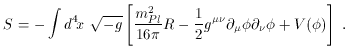(9)

Here R is the Ricci curvature scalar. Quantum fluctuations result in perturbations in the metric tensor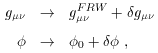(10)

where gµ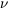FRW is the Friedmann-Robertson-Walker metric, and0 (t) is the classical solution for the homogeneous, isotropic evolution of the inflaton. The action describing the dynamics of the small perturbations can be written as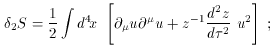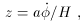(11)

i.e., the action in conformal time(d2 = a2 (t) dt2) for a scalar field in Minkowski space, with mass-squared mu2 = -z-1 d2 z / d2. Here, the scalar field u is a combination of metric fluctuationsgµand scalar field fluctuations. This scalar field is related to the amplitude of the density perturbation.

The simple matter of calculating the perturbation spectrum for a noninteracting scalar field in Minkowski space will give the amplitude and spectrum of the density perturbations. The problem is that the solution to the field equations depends upon the background field evolution through the dependence of the mass of the field upon z. Different choices for the inflaton potential V() results in different background field evolutions, and hence, different spectra and amplitudes for the density perturbations.

Before proceeding, now is a useful time to remark that in addition to scalar density perturbations, there are also fluctuations in the transverse, traceless component of the spatial part of the metric. These fluctuations (known as tensor fluctuations) can be thought of as a background of gravitons.

Although the scalar and tensor spectra depend upon V(), for most potentials they can be characterized by QRMSPS (the amplitude of the scalar and tensor spectra on large length scales added in quadrature), n (the scalar spectral index describing the best power-law fit of the primordial scalar spectrum), r (the ratio of the tensor-to-scalar contribution to C2 in the angular power spectrum), and nT ( the tensor spectral index describing the best power-law fit of the primordial tensor spectrum). For single-field, slow-roll inflation models, there is a relationship between nT and r, so in fact there are only three independent variables. Furthermore, the amplitude of the fluctuations often depends upon a free parameter in the potential, and the spectra are normalized by QRMSPS. This leads to a characterization of a wide-range of inflaton potentials in terms of two numbers, n and r.

In addition to the primordial spectrum characterized by n and r, in order to compare to data it is necessary to specify cosmological parameters (H0, the present expansion rate;0, the ratio of the present mass-energy density to the critical density - a spatially flat universe has0 = 1;B, the ratio of the present baryon density to the critical density;DM the ratio of the present dark-matter density to the critical density; and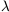, the value of the cosmological constant), as well as the nature of the dark matter.

The specification of the dark matter is by how ``hot'' the dark matter was when the universe first became matter dominated. If the dark matter was really slow at that time, then it is referred to as cold dark matter. If the dark matter was reasonably hot when the universe became matter dominated, then it is called hot dark matter. Finally, the intermediate case is called warm dark matter. Neutrinos with a mass in the range 1 eV to a few dozen eV would be hot dark matter. Light gravitinos, as appear in gauge-mediated supersymmetry breaking schemes, is an example of warm dark matter. By far the most popular dark matter candidate is cold dark matter. Examples of cold dark matter are neutralinos and axions.